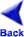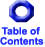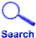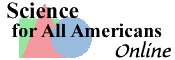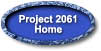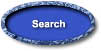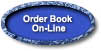## Chapter 2: THE NATURE OF MATHEMATICS

### Chapter 2: THE NATURE OF MATHEMATICS

Mathematics relies on both logic and creativity, and it is pursued both for a variety of practical purposes and for its intrinsic interest. For some people, and not only professional mathematicians, the essence of mathematics lies in its beauty and its intellectual challenge. For others, including many scientists and engineers, the chief value of mathematics is how it applies to their own work. Because mathematics plays such a central role in modern culture, some basic understanding of the nature of mathematics is requisite for scientific literacy. To achieve this, students need to perceive mathematics as part of the scientific endeavor, comprehend the nature of mathematical thinking, and become familiar with key mathematical ideas and skills.

This chapter focuses on mathematics as part of the scientific endeavor and then on mathematics as a process, or way of thinking. Recommendations related to mathematical ideas are presented in Chapter 9, The Mathematical World, and those on mathematical skills are included in Chapter 12, Habits of Mind.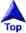### PATTERNS AND RELATIONSHIPS

Mathematics is the science of patterns and relationships. As a theoretical discipline, mathematics explores the possible relationships among abstractions without concern for whether those abstractions have counterparts in the real world. The abstractions can be anything from strings of numbers to geometric figures to sets of equations. In addressing, say, "Does the interval between prime numbers form a pattern?" as a theoretical question, mathematicians are interested only in finding a pattern or proving that there is none, but not in what use such knowledge might have. In deriving, for instance, an expression for the change in the surface area of any regular solid as its volume approaches zero, mathematicians have no interest in any correspondence between geometric solids and physical objects in the real world.

A central line of investigation in theoretical mathematics is identifying in each field of study a small set of basic ideas and rules from which all other interesting ideas and rules in that field can be logically deduced. Mathematicians, like other scientists, are particularly pleased when previously unrelated parts of mathematics are found to be derivable from one another, or from some more general theory. Part of the sense of beauty that many people have perceived in mathematics lies not in finding the greatest elaborateness or complexity but on the contrary, in finding the greatest economy and simplicity of representation and proof. As mathematics has progressed, more and more relationships have been found between parts of it that have been developed separately—for example, between the symbolic representations of algebra and the spatial representations of geometry. These cross-connections enable insights to be developed into the various parts; together, they strengthen belief in the correctness and underlying unity of the whole structure.

Mathematics is also an applied science. Many mathematicians focus their attention on solving problems that originate in the world of experience. They too search for patterns and relationships, and in the process they use techniques that are similar to those used in doing purely theoretical mathematics. The difference is largely one of intent. In contrast to theoretical mathematicians, applied mathematicians, in the examples given above, might study the interval pattern of prime numbers to develop a new system for coding numerical information, rather than as an abstract problem. Or they might tackle the area/volume problem as a step in producing a model for the study of crystal behavior.

The results of theoretical and applied mathematics often influence each other. The discoveries of theoretical mathematicians frequently turn out—sometimes decades later—to have unanticipated practical value. Studies on the mathematical properties of random events, for example, led to knowledge that later made it possible to improve the design of experiments in the social and natural sciences. Conversely, in trying to solve the problem of billing long-distance telephone users fairly, mathematicians made fundamental discoveries about the mathematics of complex networks. Theoretical mathematics, unlike the other sciences, is not constrained by the real world, but in the long run it contributes to a better understanding of that world.### MATHEMATICS, SCIENCE, AND TECHNOLOGY

Because of its abstractness, mathematics is universal in a sense that other fields of human thought are not. It finds useful applications in business, industry, music, historical scholarship, politics, sports, medicine, agriculture, engineering, and the social and natural sciences. The relationship between mathematics and the other fields of basic and applied science is especially strong. This is so for several reasons, including the following:

• The alliance between science and mathematics has a long history, dating back many centuries. Science provides mathematics with interesting problems to investigate, and mathematics provides science with powerful tools to use in analyzing data. Often, abstract patterns that have been studied for their own sake by mathematicians have turned out much later to be very useful in science. Science and mathematics are both trying to discover general patterns and relationships, and in this sense they are part of the same endeavor.
• Mathematics is the chief language of science. The symbolic language of mathematics has turned out to be extremely valuable for expressing scientific ideas unambiguously. The statement that a=F/m is not simply a shorthand way of saying that the acceleration of an object depends on the force applied to it and its mass; rather, it is a precise statement of the quantitative relationship among those variables. More important, mathematics provides the grammar of science—the rules for analyzing scientific ideas and data rigorously.
• Mathematics and science have many features in common. These include a belief in understandable order; an interplay of imagination and rigorous logic; ideals of honesty and openness; the critical importance of peer criticism; the value placed on being the first to make a key discovery; being international in scope; and even, with the development of powerful electronic computers, being able to use technology to open up new fields of investigation.
• Mathematics and technology have also developed a fruitful relationship with each other. The mathematics of connections and logical chains, for example, has contributed greatly to the design of computer hardware and programming techniques. Mathematics also contributes more generally to engineering, as in describing complex systems whose behavior can then be simulated by computer. In those simulations, design features and operating conditions can be varied as a means of finding optimum designs. For its part, computer technology has opened up whole new areas in mathematics, even in the very nature of proof, and it also continues to help solve previously daunting problems.### MATHEMATICAL INQUIRY

Using mathematics to express ideas or to solve problems involves at least three phases: (1) representing some aspects of things abstractly, (2) manipulating the abstractions by rules of logic to find new relationships between them, and (3) seeing whether the new relationships say something useful about the original things.

#### Abstraction and Symbolic Representation

Mathematical thinking often begins with the process of abstraction—that is, noticing a similarity between two or more objects or events. Aspects that they have in common, whether concrete or hypothetical, can be represented by symbols such as numbers, letters, other marks, diagrams, geometrical constructions, or even words. Whole numbers are abstractions that represent the size of sets of things and events or the order of things within a set. The circle as a concept is an abstraction derived from human faces, flowers, wheels, or spreading ripples; the letter A may be an abstraction for the surface area of objects of any shape, for the acceleration of all moving objects, or for all objects having some specified property; the symbol + represents a process of addition, whether one is adding apples or oranges, hours, or miles per hour. And abstractions are made not only from concrete objects or processes; they can also be made from other abstractions, such as kinds of numbers (the even numbers, for instance).

Such abstraction enables mathematicians to concentrate on some features of things and relieves them of the need to keep other features continually in mind. As far as mathematics is concerned, it does not matter whether a triangle represents the surface area of a sail or the convergence of two lines of sight on a star; mathematicians can work with either concept in the same way. The resulting economy of effort is very useful—provided that in making an abstraction, care is taken not to ignore features that play a significant role in determining the outcome of the events being studied.

#### Manipulating Mathematical Statements

After abstractions have been made and symbolic representations of them have been selected, those symbols can be combined and recombined in various ways according to precisely defined rules. Sometimes that is done with a fixed goal in mind; at other times it is done in the context of experiment or play to see what happens. Sometimes an appropriate manipulation can be identified easily from the intuitive meaning of the constituent words and symbols; at other times a useful series of manipulations has to be worked out by trial and error.

Typically, strings of symbols are combined into statements that express ideas or propositions. For example, the symbol A for the area of any square may be used with the symbol s for the length of the square's side to form the proposition A = s2. This equation specifies how the area is related to the side—and also implies that it depends on nothing else. The rules of ordinary algebra can then be used to discover that if the length of the sides of a square is doubled, the square's area becomes four times as great. More generally, this knowledge makes it possible to find out what happens to the area of a square no matter how the length of its sides is changed, and conversely, how any change in the area affects the sides.

Mathematical insights into abstract relationships have grown over thousands of years, and they are still being extended—and sometimes revised. Although they began in the concrete experience of counting and measuring, they have come through many layers of abstraction and now depend much more on internal logic than on mechanical demonstration. In a sense, then, the manipulation of abstractions is much like a game: Start with some basic rules, then make any moves that fit those rules—which includes inventing additional rules and finding new connections between old rules. The test for the validity of new ideas is whether they are consistent and whether they relate logically to the other rules.

#### Application

Mathematical processes can lead to a kind of model of a thing, from which insights can be gained about the thing itself. Any mathematical relationships arrived at by manipulating abstract statements may or may not convey something truthful about the thing being modeled. For example, if 2 cups of water are added to 3 cups of water and the abstract mathematical operation 2+3 = 5 is used to calculate the total, the correct answer is 5 cups of water. However, if 2 cups of sugar are added to 3 cups of hot tea and the same operation is used, 5 is an incorrect answer, for such an addition actually results in only slightly more than 4 cups of very sweet tea. The simple addition of volumes is appropriate to the first situation but not to the second—something that could have been predicted only by knowing something of the physical differences in the two situations. To be able to use and interpret mathematics well, therefore, it is necessary to be concerned with more than the mathematical validity of abstract operations and to also take into account how well they correspond to the properties of the things represented.

Sometimes common sense is enough to enable one to decide whether the results of the mathematics are appropriate. For example, to estimate the height 20 years from now of a girl who is 5' 5" tall and growing at the rate of an inch per year, common sense suggests rejecting the simple "rate times time" answer of 7' 1" as highly unlikely, and turning instead to some other mathematical model, such as curves that approach limiting values. Sometimes, however, it may be difficult to know just how appropriate mathematical results are—for example, when trying to predict stock-market prices or earthquakes.

Often a single round of mathematical reasoning does not produce satisfactory conclusions, and changes are tried in how the representation is made or in the operations themselves. Indeed, jumps are commonly made back and forth between steps, and there are no rules that determine how to proceed. The process typically proceeds in fits and starts, with many wrong turns and dead ends. This process continues until the results are good enough.

But what degree of accuracy is good enough? The answer depends on how the result will be used, on the consequences of error, and on the likely cost of modeling and computing a more accurate answer. For example, an error of 1 percent in calculating the amount of sugar in a cake recipe could be unimportant, whereas a similar degree of error in computing the trajectory for a space probe could be disastrous. The importance of the "good enough" question has led, however, to the development of mathematical processes for estimating how far off results might be and how much computation would be required to obtain the desired degree of accuracy.